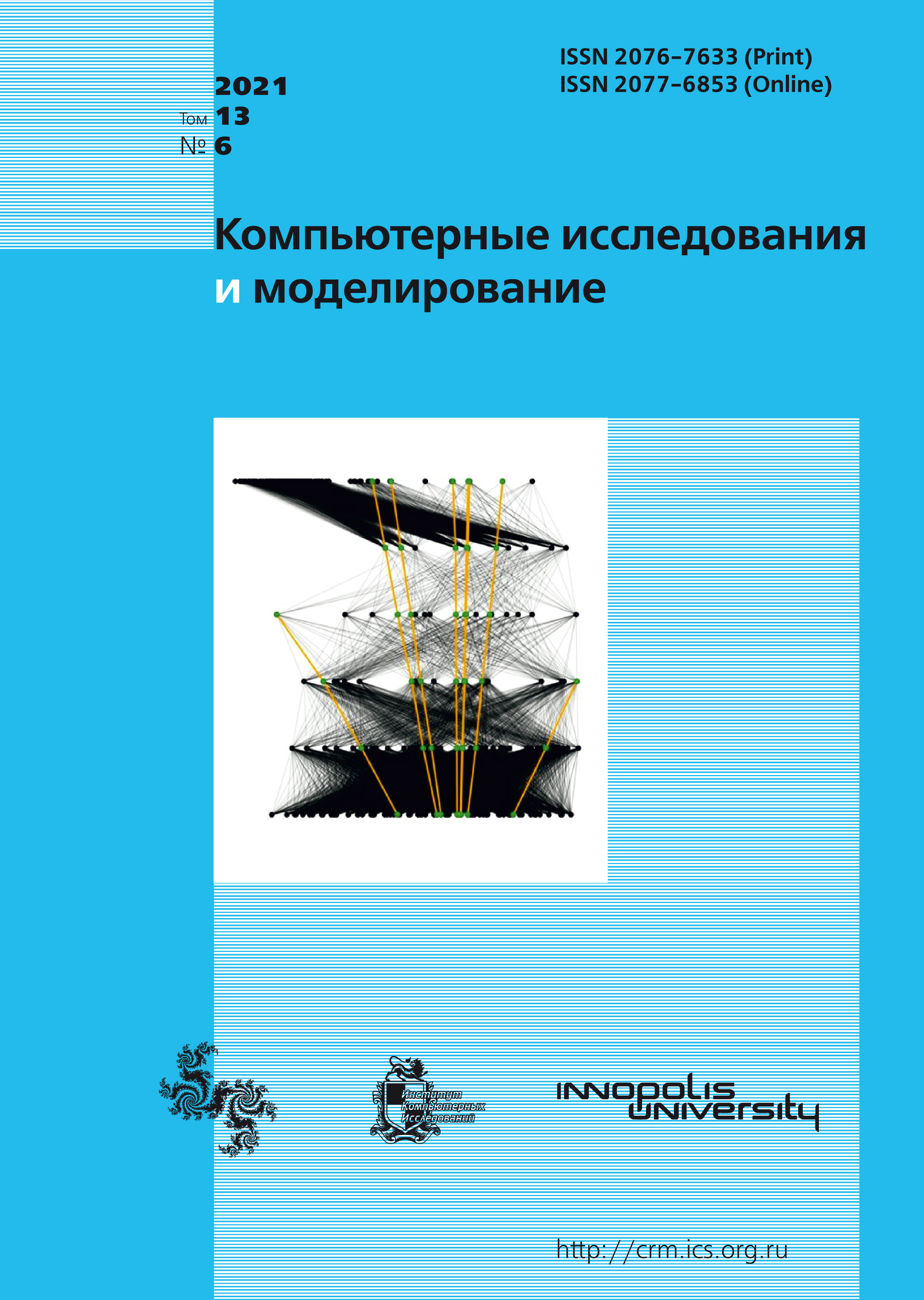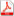Latest issue Issue 6, 2021 Vol. 13

All issues

Numerical simulation of flow in a two-dimensional flat diffuser based on two fluid turbulence modelspdf (891K)

The article presents the results of a numerical study of the flow structure in a two-dimensional flat diffuser. A feature of diffusers is that they have a complex anisotropic turbulent flow, which occurs due to recirculation flows. The turbulent RANS models, which are based on the Boussinesq hypothesis, are not able to describe the flow in diffusers with sufficient accuracy. Because the Boussinesq hypothesis is based on isotropic turbulence. Therefore, to calculate anisotropic turbulent flows, models are used that do not use this hypothesis. One of such directions in turbulence modeling is the methods of Reynolds stresses. These methods are complex and require rather large computational resources. In this work, a relatively recently developed two-fluid turbulence model was used to study the flow in a flat diffuser. This model is developed on the basis of a two-fluid approach to the problem of turbulence. In contrast to the Reynolds approach, the two-fluid approach allows one to obtain a closed system of turbulence equations using the dynamics of two fluids. Consequently, if empirical equations are used in RANS models for closure, then in the two-fluid model the equations used are exact equations of dynamics. One of the main advantages of the two-fluid model is that it is capable of describing complex anisotropic turbulent flows. In this work, the obtained numerical results for the profiles of the longitudinal velocity, turbulent stresses in various sections of the channel, as well as the friction coefficient are compared with the known experimental data. To demonstrate the advantages of the used turbulence model, the numerical results of the Reynolds stress method EARSM are also presented. For the numerical implementation of the systems of equations of the two-fluid model, a non-stationary system of equations was used, the solution of which asymptotically approached the stationary solution. For this purpose, a finite-difference scheme was used, where the viscosity terms were approximated by the central difference implicitly, and for the convective terms, an explicit scheme against the flow of the second order of accuracy was used. The results are obtained for the Reynolds number Re = 20 000. It is shown that the two-fluid model, despite the use of a uniform computational grid without thickening near the walls, is capable of giving a more accurate solution than the rather complex Reynolds stress method with a high resolution of computational grids.

Keywords: Navier – Stokes equations, diffuser, disruptive flow, two-dimensional model, control volume method, turbulent voltages
Citation in English: Malikov Z.M., Madaliev M.E. Numerical simulation of flow in a two-dimensional flat diffuser based on two fluid turbulence models // Computer Research and Modeling, 2021, vol. 13, no. 6, pp. 1149-1160
Citation in English: Malikov Z.M., Madaliev M.E. Numerical simulation of flow in a two-dimensional flat diffuser based on two fluid turbulence models // Computer Research and Modeling, 2021, vol. 13, no. 6, pp. 1149-1160
DOI: 10.20537/2076-7633-2021-13-6-1149-1160

Full-text version of the journal is also available on the web site of the scientific electronic library eLIBRARY.RU

The journal is included in the Russian Science Citation Index

The journal is included in the List of Russian peer-reviewed journals publishing the main research results of PhD and doctoral dissertations.

International Interdisciplinary Conference "Mathematics. Computing. Education"

The journal is included in the RSCI

Indexed in Scopus